# 继承与派生——访问控制

## 一.知识要点

（一）知识回顾：

（二）知识引入：

• 派生类的新增成员访问从基类继承的成员
• 派生类外部（非类族内的成员），通过派生类的对象访问从基类继承的成员

## 二.公有继承

（一）知识要点：
1.当类的继承方式为公有继承时，基类的公有成员和保护成员仍作为派生类的公有成员和保护成员，派生类的其他成员可以直接访问他们。
2.在类之外只能通过派生类的对象访问从基类继承的公有成员。

(二)代码分析
（教材256页）

#include <iostream>
using namespace std;

class Point{
public:
void initPoint(float x=0,float y=0)
{
this->x=x;
this->y=y;
}//构造函数
void move(float movex,float movey)
{
x=x+movex;
y=y+movey;
}
float getx() const {return x;}
float gety() const {return y;}
private:
float x,y;
};

class Rectangle:public Point//派生类的定义
{
public:
void initRectangle(float x,float y,float w,float h)
{
this->w=w;
this->h=h;
initPoint(x,y);//直接使用基类的公有成员函数
}
float geth() const {return h;}
float getw() const {return w;}
private:
float w,h;
};

int main()
{
Rectangle rect;//定义Rectangle类的对象
rect.initPoint(6,6);
cout<<rect.getx()<<endl;
cout<<rect.gety()<<endl;
cout<<"--------"<<endl;

rect.initRectangle(1,2,3,4);
rect.move(2,1);
cout<<rect.getx()<<endl;
cout<<rect.gety()<<endl;
cout<<rect.geth()<<endl;
cout<<rect.getw()<<endl;
return 0;
}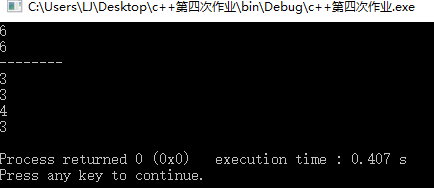1.修改代码：这里我们让initpoint函数的属性是protected，同时在主函数中不让对象rect调用。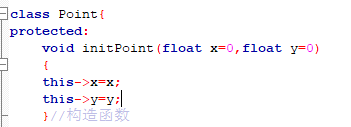2.修改代码:这里我们让initpoint函数的属性是protected，同时不改变原始的主函数，即让对象rect调用基类里的两个函数。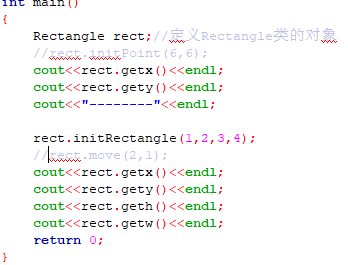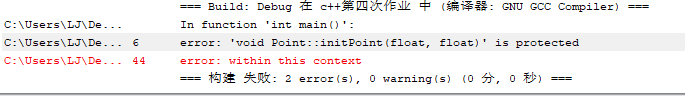## 三.私有继承

（一）知识要点：
1.当类的继承方式为私有继承的时候，基类中的公有成员和保护成员都以私有成员的身份出现在派生类中，派生类的其他成员可以直接访问基类中的公有成员和保护成员。
2.在类族外部通过派生类的对象无法直接基类的公有成员，保护成员和私有成员

(二)代码分析
（以下代码非教材上的代码，教材上私有继承的代码请参考256页）

#include <iostream>
using namespace std;

class point
{
private:
int a;
public:
void inita(int x){a=x;}
int geta(){return a;}
};

class p:private point
{
private:
int b;//派生类的私有数据成员
public:
void init(int x,int y)
{
b=y;
inita(x);//调用基类的成员函数
}
int getb(){return b*geta();}//调用基类的成员函数
};

int main()
{
p op;//创建派生类对象
op.init(2,3);
cout<<op.getb()<<endl;
return 0;
}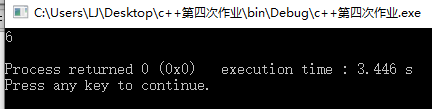1.修改代码：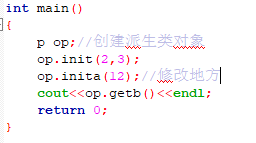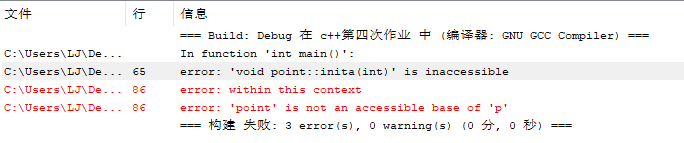2.修改代码：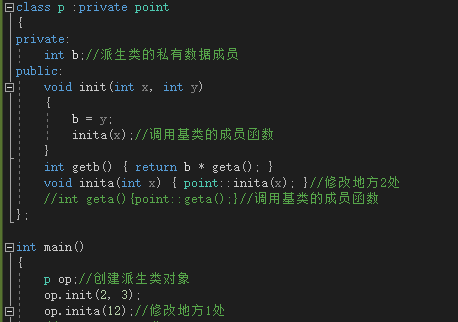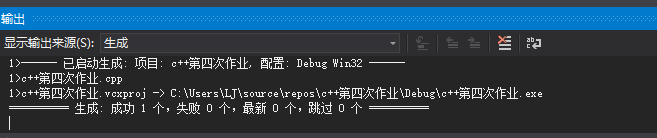3.修改代码：

#include"pch.h"
#include <iostream>

using namespace std;

class point
{
private:
int a;
public:
void inita(int x) { a = x; }
int geta() { return a; }
};

class p :private point
{
private:
int b;//派生类的私有数据成员
public:
void init(int x, int y)
{
b = y;
inita(x);//调用基类的成员函数
}
int getb() { return b * geta(); }

void inita(int x) { point::inita(x); }//修改地方2处
int geta(){ return point::geta();}//调用基类的成员函数
};

int main()
{
p op;//创建派生类对象
op.init(2, 3);
op.inita(12);//修改地方1处
cout<<op.getb()<<endl;
cout<<op.geta()<<endl;
return 0;
}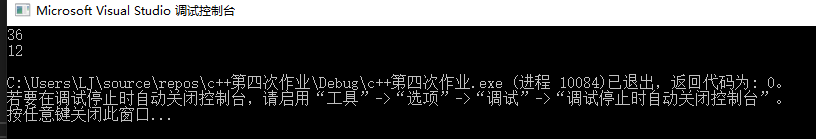## 四.保护继承

（一）知识要点：
1.当类的继承方式为保护继承的时候，基类中的公有成员和保护成员都以宝华成员的身份出现在派生类中，派生类的其他成员可以直接访问基类中的公有成员和保护成员。
2.在类的外部通过派生类的对象无法直接访问它们。

(二)代码分析


#include"pch.h"
#include <iostream>

using namespace std;

class point
{
private:
int a;
public:
void inita(int x) { a = x; }
int geta() { return a; }
};

class p :protected point//保护继承
{
private:
int b;//派生类的私有数据成员
public:
void init(int x, int y)
{
b = y;
inita(x);//调用基类的成员函数
}
int getb() { return b * geta(); }

void inita(int x) { point::inita(x); }
int geta(){ return point::geta();}//调用基类的成员函数
};

int main()
{
p op;//创建派生类对象
op.init(3, 4);
cout<<op.getb()<<endl;
op.inita(6);
cout<<op.geta()<<endl;
return 0;
}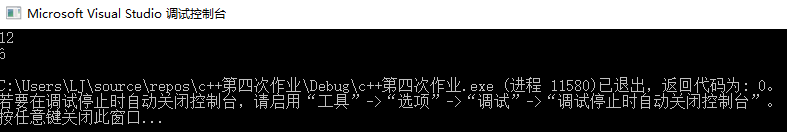## 五.知识点汇总

• 基类成员对派生类的可见性

public protected private

• 基类成员对派生类对象的可见性

public protected private

 问题思考： 在私有继承和保护继承下，基类的所有成员在派生类中或派生类对象中的访问属性都是相同的，那么两者到底有什么区别？

（a)当B私有继承A的时候，在B类中A类的所有成员都将是私有的了，因此无论C以后再以什么方式来继承B中的成员，在C类中都将无法访问到A类中的成员了。
(b)当B保护继承A时，在B类中A类的公有成员和保护成员都将是保护成员了，这样无论C是以什么方式来继承B中的成员，在C类中都可以访问A中的公有成员和保护成员。

posted on 2019-10-12 17:30  咬一口云朵软绵绵  阅读(164)  评论(0编辑  收藏  举报